# UIUCTF 2022 - Pierated Art

##### by not_really

“Composition with Red, Yellow, and Blue by Piet Mondrian, with red graffiti all over it”

Downloaded some art from a sketchy torrent provider (piet_pirate), and there are scribbles all over it.

Update: The passwords (not the flag) are in lowercase ASCII

`\$ nc pierated-art.chal.uiuc.tf 1337`

author: spicypete, richyliu

## First look

Opening netcat to the server displays this:

``````== proof-of-work: disabled ==
Torrented Picture Data (Base64):
iVBORw0KGgoAAAANSUhEUgAAAyYAAAQACAIAAACbB9rAAA... (long base 64 string)
Enter flag #1/10 (15s):
``````

Decoding that manually gives us a very large image: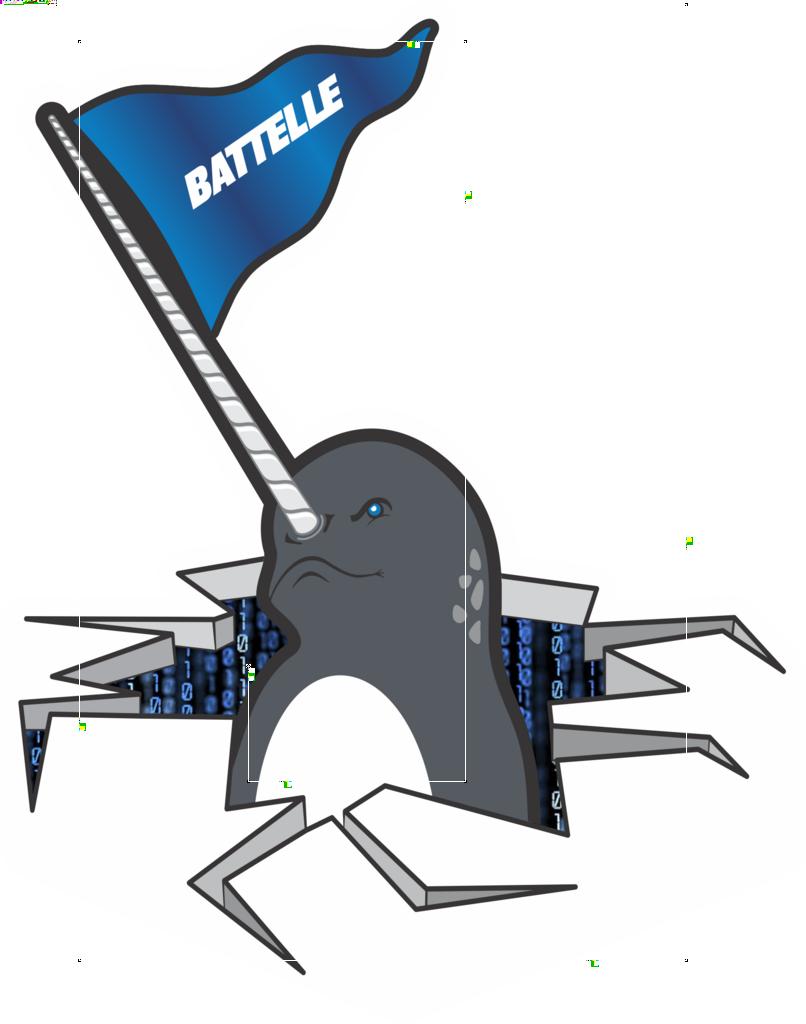Zoomed into the top left: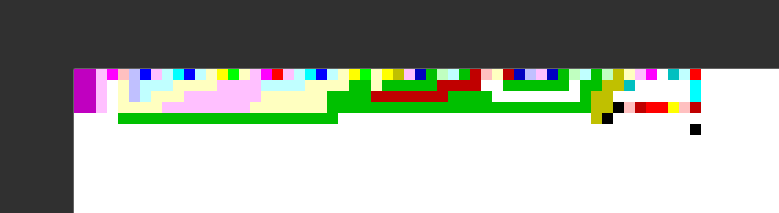I’d seen an esoteric language that used colors like this, and a search brought me to Piet on Wikipedia. Esolangs.org has more info. The TLDR:

• A codel is the unit of measurement, and in our case, the same as one pixel. If we zoomed in the image 200%, the codel size would be 2 (2x2 block of pixels per codel).
• The command executed by each pixel is based on the relative hue and lightness of the previous pixel.
• The direction pointer is the pixel that is being executed and the direction to execute for the next one. Essentially, it starts in the top left corner and goes right until it hits a wall or rotates with the `pointer` command.
• Black pixels make the direction pointer rotate clockwise. White pixels are skipped.

There are a bunch of different commands as well, but not all of them are used so I’ll only go over those ones when I get to those parts.

## Digging in

The first thing I wanted was some kind of “disassembler” or “debugger” so I could understand what was going on. Other players found npiet and repiet command line tools which were able to disassemble pretty well.

Here’s some output from npiet trace (again, I did not find this until after the competition end):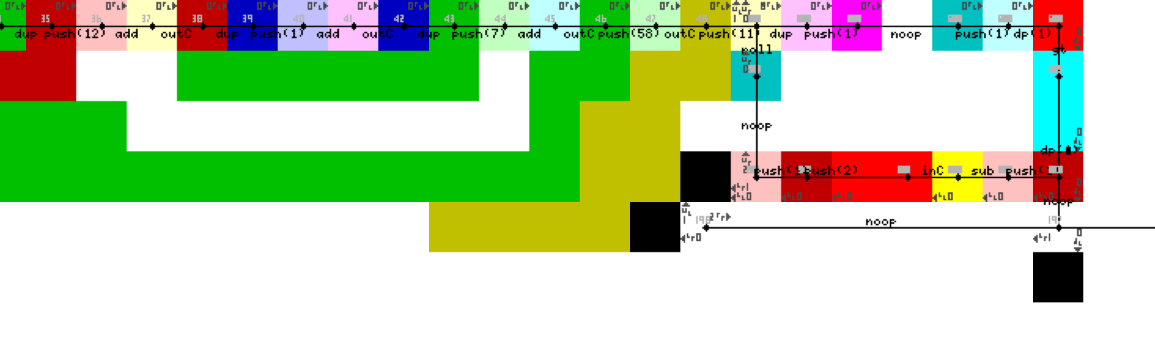While I was doing the challenge, I didn’t find this or any other tools that could open slightly big images (806 x 1024). The first tool I came across that could open it was Pietron (Piet in Electron) but it was extremely laggy and took ages to open an image. However, it was based off of a different program, Pidet (written in C#) which seemed to perform decently.

## Debugging with Pidet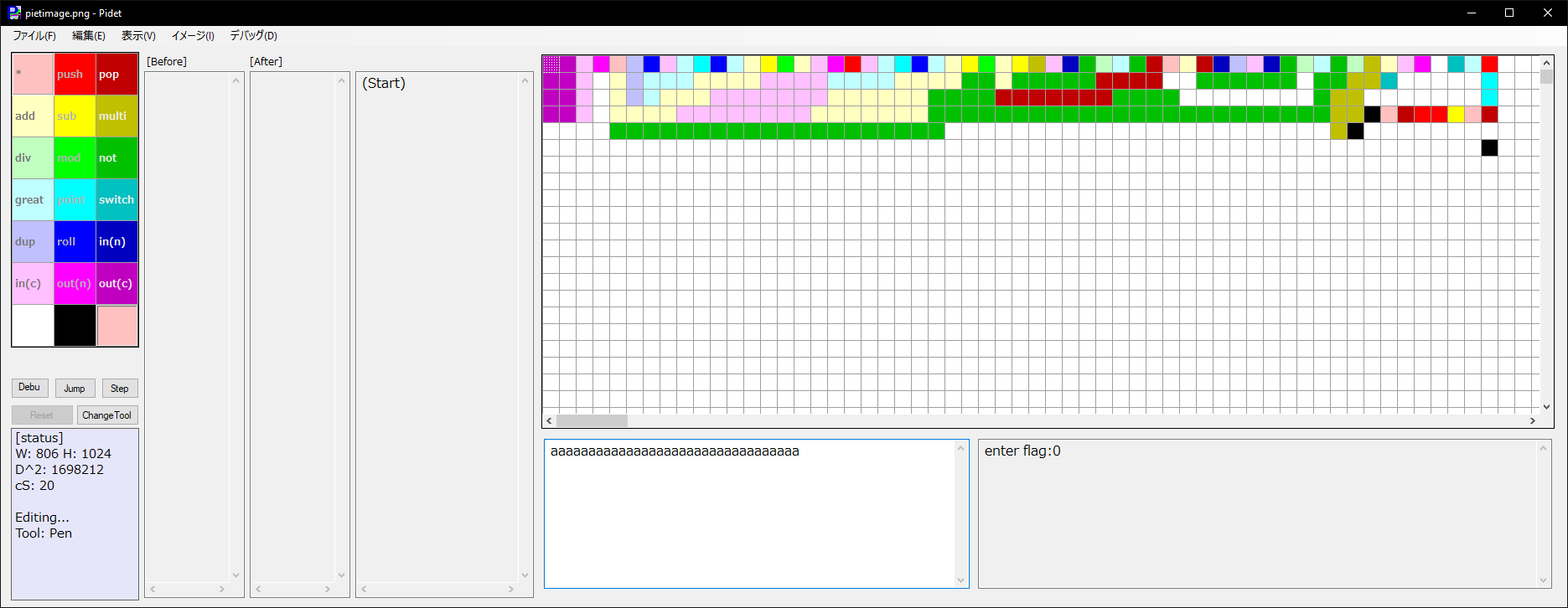Pressing F5 prints `enter flag:` and asks for input. Pidet will complain there is no input if you haven’t typed anything into the input box, so I filled it with `a`s to make it happy.

After running the input, it prints a 0, probably for “the flag is incorrect”. I tried following the pointer for a while, but the program doesn’t automatically scroll to it which meant I had to keep scrolling over to find where the pointer was.

At this point, I realized I could just simplify the image down to a much smaller one which would’ve worked in the other tools that were struggling to open the large file 🤦: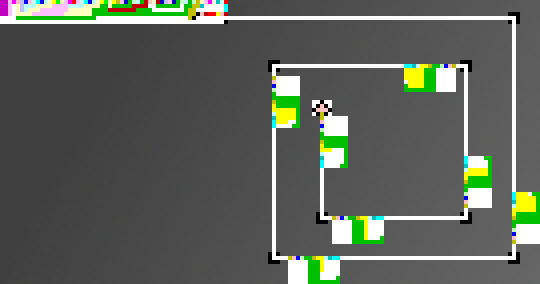In any case, I added a textbox into Pidet and added print statements on each command to log everything being run. Here’s what the above program looks like:

``````# print "enter flag:"
push(8)
push(4)
multi(8, 4) == 32
dup()
push(3)
multi(32, 3) == 96
dup()
push(5)
out(c)
dup()
push(14)
# snip ...
out(c)
push(58)
out(c)
push(8)
dup()
push(1)
push(1)
point() == 1
great(8, 1) == (true)
point() == 2
push(1)
sub(8, 1) == 7
in(c)
push(2)
push(1)
roll()
dup()
push(1)
push(1)
point() == 1
great(7, 1) == (true)
point() == 2
push(1)
sub(7, 1) == 6
in(c)
push(2)
push(1)
roll()
dup()
push(1)
push(1)
# snip ...
point() == 1
great(1, 1) == (false)
point() == 1
# exit input loop
# check first character
push(2)
push(1)
roll()
push(25)
push(26)
mod(122, 26) == 18
not(18) == (0)
multi(1, 0) == 0
# check next character
push(2)
push(1)
roll()
push(5)
push(26)
mod(102, 26) == 24
not(24) == (0)
multi(0, 0) == 0
# ...
push(2)
push(1)
roll()
push(20)
push(26)
mod(117, 26) == 13
not(13) == (0)
multi(0, 0) == 0
push(2)
push(1)
roll()
push(25)
push(26)
mod(122, 26) == 18
not(18) == (0)
multi(0, 0) == 0
push(2)
push(1)
roll()
push(12)
push(26)
mod(109, 26) == 5
not(5) == (0)
multi(0, 0) == 0
push(2)
push(1)
roll()
push(7)
push(26)
mod(104, 26) == 0
not(0) == (1)
multi(0, 1) == 0
push(2)
push(1)
roll()
push(4)
push(26)
mod(101, 26) == 23
not(23) == (0)
multi(0, 0) == 0
out(n)
``````

Whenever the “input loop” in the top left exits, it reads only 7 characters. There are also only 7 boxes before the program reaches the end. So each box is probably checking a single character.

A single check looks like this (my input was all `a`s so the input should be any number with `97` in it):

``````push(2)
push(1)
roll()
push(25)
push(26)
mod(122, 26) == 18
not(18) == (0)
multi(1, 0) == 0
``````

This is what that would be in pseudo code:

``````# not in the above code, but it's set
# to 1 before the first character check
some_number = 1

# check first character
t0 = input + 25
t1 = t0 % 26
t2 = 1 if t1 == 0 else 0
some_number *= t2
# check second character
t0 = input + 5
t1 = t0 % 26
t2 = t1 == 0
some_number *= t2
``````

That `some_number` value is the value printed at the end with a 0 or 1. So to decode this we just need to know to turn `((input + x) % 26) == 0` into a character list. I started by making a list by hand of uppercase letters:

`NMLKJIHGFEDCBAZYXWVUTSRQPO`

For example, if the program was adding 10, input == 0x44 would solve the above equation which is `D` in ASCII. This list makes it easy to access the 10th element to get `D`.

It turns out that the server doesn’t accept uppercase, only lowercase (this was before the challenge description was updated to say only lowercase.) So I had to switch to a lowercase list instead:

`hgfedcbazyxwvutsrqponmlkji`

Doing this by hand for the example, I got `icnivad`. This didn’t work in the Pidet, but I didn’t realize that because of how the input is read onto the stack, it’s reversed. So the real answer is `davinci`. That makes a lot more sense now!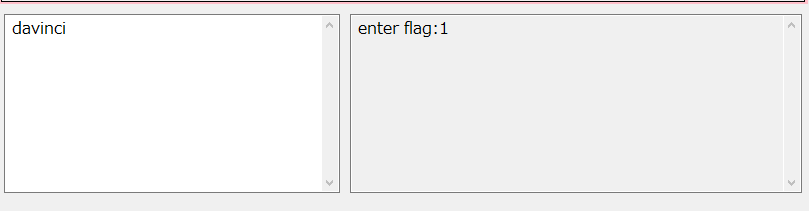## Scripting it

The server requires you to solve all flags in under 15 seconds so there’s no way I could do it by hand. I would need to find a way to script it.

Like I said before, most people chose to use npiet or repiet to script their solutions. However, I decided “f*** it, let’s just build one from scratch.” It doesn’t have to be fully accurate since there are noticeable patterns in the image.

• The positions of the “blocks” are randomized, so we’ll have to emulate the pointer.
• Every block is basically the same except the direction it faces and the amount of yellow codels which are the value used for the `add` command. We can just count the amount of yellow pixels around the current pixel.
• The ending is reached when there is a pink pixel on the left, top, and right of the pointer.

For counting yellow pixels, we can just select a block of pixels configured for each of the four directions instead of “flood filling” to find the amount of touching yellow pixels.

Region of yellow pixels to count for "block" in right direction (bottom left)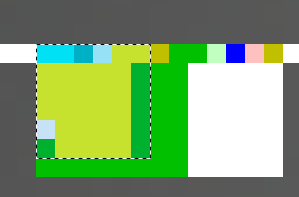Region of pixels to count for "block" in downwards direction (top right)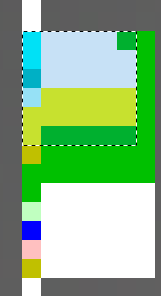Region of pixels to count for "block" in left direction (bottom right)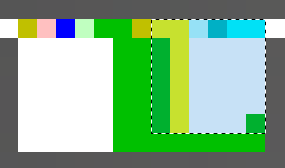Region of pixels to count for "block" in upwards direction (also bottom right)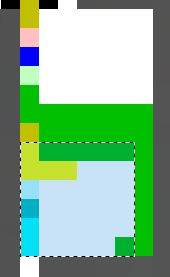## Solution script

``````from PIL import Image
import base64
import io
from pwn import *

class PietSim():
def reset(self, img):
self.posX = 49
self.posY = 4
self.dir = 0
self.last_color = (0,0,0)
self.result = ""
self.img:Image = img
self.char_map = "hgfedcbazyxwvutsrqponmlkjihg"

def move(self, times):
if self.dir == 0: # right
self.posX += times
elif self.dir == 1: # down
self.posY += times
elif self.dir == 2: # left
self.posX -= times
else: # up
self.posY -= times

def rotate(self, times):
self.dir = (self.dir + times) % 4

def count_yellow_pixels(self):
count = 0
# the yellow pixels can fit in a 5x5 box + the two on the line
# note these are relative from the first encountered pixel,
# not the second one, so one of the axes will have a +/-1 offset
for i in range(6):
for j in range(6):
if self.dir == 0:
pixel = self.img.getpixel((self.posX + 1 - i, self.posY + j))
elif self.dir == 1:
pixel = self.img.getpixel((self.posX + i, self.posY + 1 - j))
elif self.dir == 2:
pixel = self.img.getpixel((self.posX - 1 + i, self.posY + j))
elif self.dir == 3:
pixel = self.img.getpixel((self.posX + i, self.posY - 1 + j))

if pixel == (255, 255, 0):
count += 1

return count

def step(self):
self.move(1)

color = self.img.getpixel((self.posX, self.posY))
if color == (0, 0, 0): # black
self.move(-1) # step back
self.rotate(1) # and rotate
elif color == (255, 255, 0): # yellow (value to be used for add)
# only use the first yellow square, but not the next one
if self.last_color != (255, 255, 0):
pixel_count = self.count_yellow_pixels()
self.result = self.char_map[pixel_count] + self.result

self.last_color = color

return self.should_exit()

def one_if_pink(self, color):
if color == (255, 192, 192):
return True
return False

def should_exit(self):
left_color = self.img.getpixel((self.posX - 1, self.posY))
right_color = self.img.getpixel((self.posX + 1, self.posY))
top_color = self.img.getpixel((self.posX, self.posY - 1))
bottom_color = self.img.getpixel((self.posX, self.posY + 1))

pink_pixel_count = 0
pink_pixel_count += self.one_if_pink(left_color)
pink_pixel_count += self.one_if_pink(right_color)
pink_pixel_count += self.one_if_pink(top_color)
pink_pixel_count += self.one_if_pink(bottom_color)

# 3 out of the 4 directions need to have pink
return pink_pixel_count >= 3

def solve(b64):
data = base64.b64decode(b64)
stream = io.BytesIO(data)
img = Image.open(stream)

ps = PietSim()
ps.reset(img)

while True:
if ps.step():
break

return ps.result

p = remote("pierated-art.chal.uiuc.tf", 1337)
for i in range(10):
print(p.recvuntil(b"Torrented Picture Data (Base64):\n"))

b64 = p.recvline()[:-1]
solution = solve(b64)

print(p.recvuntil(b" (15s):"))
p.send(solution.encode("utf-8") + b"\n")

p.interactive()

solve()
``````
``````potato@pop-os:~/Desktop\$ python3 solvepiet.py
[+] Opening connection to pierated-art.chal.uiuc.tf on port 1337: Done
b'== proof-of-work: disabled ==\nTorrented Picture Data (Base64):\n'
b'Enter flag #1/10 (15s):'
b'Correct!\nTorrented Picture Data (Base64):\n'
b'Enter flag #2/10 (15s):'
b'Correct!\nTorrented Picture Data (Base64):\n'
b'Enter flag #3/10 (15s):'
b'Correct!\nTorrented Picture Data (Base64):\n'
b'Enter flag #4/10 (15s):'
b'Correct!\nTorrented Picture Data (Base64):\n'
b'Enter flag #5/10 (15s):'
b'Correct!\nTorrented Picture Data (Base64):\n'
b'Enter flag #6/10 (15s):'
b'Correct!\nTorrented Picture Data (Base64):\n'
b'Enter flag #7/10 (15s):'
b'Correct!\nTorrented Picture Data (Base64):\n'
b'Enter flag #8/10 (15s):'
b'Correct!\nTorrented Picture Data (Base64):\n'
b'Enter flag #9/10 (15s):'
b'Correct!\nTorrented Picture Data (Base64):\n'
b'Enter flag #10/10 (15s):'
[*] Switching to interactive mode
Correct!
i'll just use google images next time :D
uiuctf{m0ndr14n_b3st_pr0gr4mm3r_ngl}
[*] Got EOF while reading in interactive
\$
``````